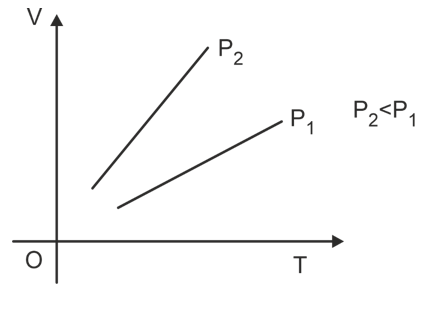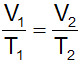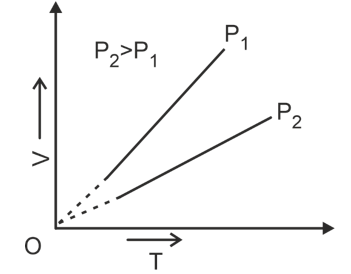# Formula For Charles Law

## Charles Law

### Definition of Charles Law

At a constant pressure, the volume of a given mass of any gas varies directly with the absolute temperature. For More Chemistry Formulas just check out main page of Physics Wallah.Mathematically: V∝T (for a given n and P)

V/T = constant

If V1 and V2 are volumes of a gas at temperature T1 and T2 respectively and the pressure is kept constant, then:Graphically it is expressed as shown in the figure. Each line is called as Isobar. Experiments have shown that when 273 ml sample of a gas at 0°C is heated to 1°C, its volume increases by 1 ml, i.e., it becomes 274 ml. At 10°C, the volume increases to 283 ml if the pressure remains constant in both cases. Similarly, when 273 ml sample of gas at 0°C is cooled to –1°C, its volume decrease to 272 ml while at -10°C the volume decreases to 263 ml if the pressure remains constant.

Thus, all gases expand or contact by the same fraction of their volumes at 0°C per degree change of temperature, i.e., for each degree change of temperature, the volume of a sample of a gas changes by the fraction 1/273 of its volume at 0°C.

Let the volume of a given amount of a gas be V0 at 0°C. The temperature is increased by t°C and the new volume becomes Vt### This is Charles law. It can be stated as follows:

The volume of a given amount of a gas at constant pressure varies directly as its absolute temperature. V∝T (if pressure is kept constant)Since volume is directly proportional to Kelvin temperature, the volume of a gas should theortically be zero at Kelvin zero. However, gases liquefy and then solidify before this low temperature is reached. In fact, no substance exists as a gas at a temperature near Kelvin zero, through the straight-line plots can be extrapolated to zero volume. The temperature that corresponds to zero volume is -273.15°C.

### Solved Examples of Charles Law

Q1. A flask is of a capacity of 1 liter. What volume of air will escape from the flask if it is heated from 270C to 370C? Assume pressure is constant.

Ans. Since pressure is constant ;  1/300=V1/310

V1 = 1.0333 litres

Since capacity is 1 liter

Thus Volume escaped = 1.0333-1 = 0.0333 L